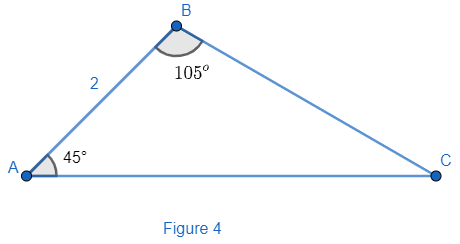QuestionAnswers

# In figure 4, if AB = 2, calculate area of $\Delta ABC.$a) 2.45b) 2.73c) 3.86d) 4.89e) 5.46Verified
114.3K+ Views
Hint: “Sum of interior angles of a triangle is ${{180}^{\circ }}$ “use this property to find out the remaining angle of $\Delta ABC$ . Now, apply the sine rule to get the required sides for calculating the area of the triangle. Sine rule is given as
$\dfrac{\sin A}{a}=\dfrac{\sin B}{b}=\dfrac{\sin C}{c}$
Where a, b, c are the sides of any triangle with A, B, C as angles opposite to sides (a, b, c) respectively. Use the given identity for calculating the area of given triangle as
Area of triangle $=\dfrac{1}{2}\times \left( a\times b \right)\sin \theta$ where (a, b) are the sides of the triangle and $\theta$ is the angle between side a and b.

Here, we have a triangle ABC with side AB of length ‘2’ units and $\angle A={{45}^{\circ }},\angle B={{105}^{\circ }}$ hence, we need to determine the area of triangle ABC.
As we know the fundamental property of a triangle that sums all the interior angles of any triangle is ${{180}^{\circ }}$ . So, we have
$\angle A+\angle B+\angle C={{180}^{\circ }}$
Put $\angle A={{45}^{\circ }},\angle B={{105}^{\circ }}$ from the given diagram in the problem. Hence, we get
\begin{align} & {{45}^{\circ }}+{{105}^{\circ }}+\angle C={{180}^{\circ }} \\ & {{150}^{\circ }}+\angle C={{180}^{\circ }} \\ & \angle C={{180}^{\circ }}-{{105}^{\circ }}={{30}^{\circ }}..................\left( i \right) \\ \end{align}
Hence, the value of $\angle {{30}^{\circ }}$ . Now, we can apply sine rule of a triangle which is given as
$\dfrac{\sin A}{a}=\dfrac{\sin B}{b}=\dfrac{\sin C}{c}...................\left( ii \right)$
Where (a, b, c) are the sides of the $\Delta ABC$ and A, B, C are the angles of $\Delta ABC$ opposite to the sides of length a, b, c respectively. Hence, on applying sine rule with the given triangle from the equation (ii), we get
$\dfrac{\sin A}{BC}=\dfrac{\sin B}{AC}=\dfrac{\sin C}{AB}$
Putting the values of $\angle A,\angle B,\angle C$ and the side AB in the above expression, we get
$\dfrac{\sin 45}{BC}=\dfrac{\sin 105}{AC}=\dfrac{\sin {{30}^{\circ }}}{2}..................\left( iii \right)$
Now, we can solve the first and last fraction of the equation (iii) to find the value of BC. So, we get
$\dfrac{\sin 45}{BC}=\dfrac{\sin 30}{2}$
Now, put the values of $\sin {{45}^{\circ }},\sin {{30}^{\circ }}$ to the above expression which are given as
$\sin {{45}^{\circ }}=\dfrac{1}{\sqrt{2}},\sin {{30}^{\circ }}=\dfrac{1}{2}$
Hence, we get
$\dfrac{\dfrac{1}{\sqrt{2}}}{BC}=\dfrac{\dfrac{1}{2}}{2}$
On cross multiplying the above equation we get
$\dfrac{2}{\sqrt{2}}=\dfrac{BC}{2}$
Hence, we get
$BC=\dfrac{4}{\sqrt{2}}$
Now, we know the area of any triangle can be given with the help of the relation $\dfrac{1}{2}\left( a\times b \right)\sin \theta$ where ‘a’ and ‘b’ are the sides of a triangle and $\theta$ is the angle between side of length ‘a’ and ‘b’. Hence, area of given triangle ABC is given as
$ar\left( ABC \right)=\dfrac{1}{2}\times AB\times BC\times \sin {{105}^{\circ }}...............\left( iv \right)$
Now, we know the values of AB and BC as 2 and $\dfrac{4}{\sqrt{2}}$ respectively. So, we need to calculate the value of $\sin {{105}^{\circ }}$ . We know the identity of $\sin \left( A+B \right)$ as
$\sin \left( A+B \right)=\sin A\cos B+\cos A\sin B............\left( v \right)$
Put $A=60,B={{45}^{\circ }}$ to the above expression we get
\begin{align} & \sin \left( 60+45 \right)=\sin {{60}^{\circ }}\cos {{45}^{\circ }}+\cos {{60}^{\circ }}\sin {{45}^{\circ }} \\ & \sin 105{{=}^{\circ }}\sin {{60}^{\circ }}\cos {{45}^{\circ }}+\cos {{60}^{\circ }}\sin {{45}^{\circ }} \\ \end{align}
Now, we know the values
$\cos {{60}^{\circ }}=\dfrac{1}{2},\sin {{60}^{\circ }}=\dfrac{\sqrt{3}}{2},\cos {{45}^{\circ }}=\sin {{45}^{\circ }}=\dfrac{1}{\sqrt{2}}$
So, we get value of $\sin {{105}^{\circ }}$ as
\begin{align} & \sin {{105}^{\circ }}=\dfrac{\sqrt{3}}{2}\times \dfrac{1}{\sqrt{2}}+\dfrac{1}{2}\times \dfrac{1}{\sqrt{2}}=\dfrac{\sqrt{3}}{2\sqrt{2}}+\dfrac{1}{2\sqrt{2}} \\ & \sin {{105}^{\circ }}=\dfrac{\sqrt{3}+1}{2\sqrt{2}}.............\left( vi \right) \\ \end{align}
Now, put the values of side AB, BC and $\sin {{105}^{\circ }}$ to the equation (iv) to get the area of $\Delta ABC$ . Hence, we get
\begin{align} & ar\left( \Delta ABC \right)=\dfrac{1}{2}\times 2\times \dfrac{4}{\sqrt{2}}\times \dfrac{\sqrt{3}+1}{2\sqrt{2}} \\ & ar\left( \Delta ABC \right)=\dfrac{4\left( \sqrt{3}+1 \right)}{4}=\sqrt{3}+1 \\ \end{align}
Now, put $\sqrt{3}=1.7325$ to the above expression. Hence, we get $ar\left( \Delta ABC \right)$ as
$ar\left( \Delta ABC \right)=1.7325+1=2.7325uni{{t}^{2}}$
So, up to two digits after decimal, we can give $ar\left( \Delta ABC \right)$ as
$ar\left( \Delta ABC \right)=2.73uni{{t}^{2}}$
Hence, option (b) is correct.

Note: One may find the sides of the given triangle with the help of cosine formula as well. It is given as
$\cos \theta =\dfrac{{{b}^{2}}+{{c}^{2}}-{{a}^{2}}}{2bc}$
Where $\theta$ is the angle between b and c and (a, b, c) are sides of the triangle. So, we need to apply the above relation twice with any two angles of triangle and proceed for calculating the area.
One may calculate area by using the Heron’s formula as well it is given as $\text{Area of triangle}=\sqrt{s\left( s-a \right)\left( s-b \right)\left( s-c \right)}$
Where $s=\dfrac{a+b+c}{2}$ and (a, b, c) are sides of the triangle. Take care while applying the sine rule in the solution. One may put the wrong angle or wrong side to anywhere in the equation of sine rule. It is given as
$\dfrac{\sin A}{a}=\dfrac{\sin B}{b}=\dfrac{\sin }{c}$
Where a, b, c are sides of triangle and A, B, C are the angles in front of sides a, b, c respectively. So, take care with the positions of sides and the angles.